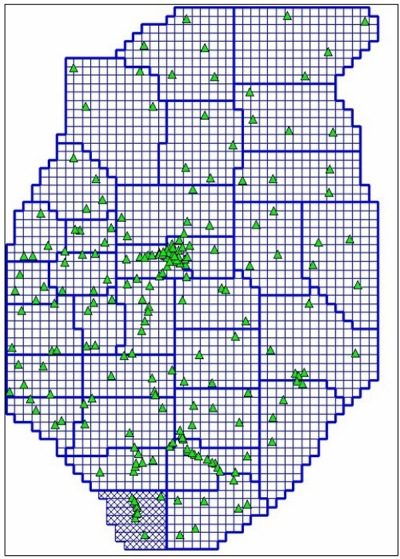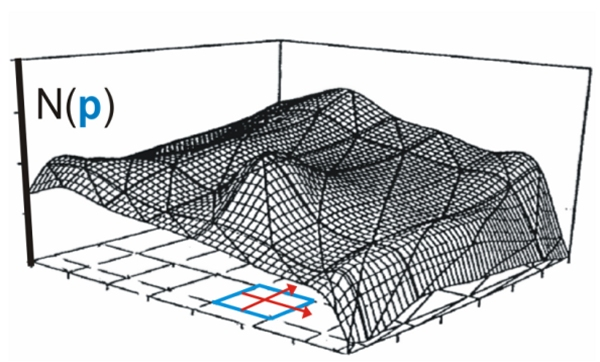### Geometrical Observation Components and Parameterization: 1st development stage of DFHRS Concept and Software (Version 4)

In the DFHRS concept at its first stage of development, a continuous polynomial surface over of a grid of finite element meshes (FEM) with polynomial parameters p (fig. 2a, thin blue lines) is used as a carrier function for the HRS. The FEM surface of the HRS is therefore called NFEM(p|B,L,h), (fig. 2b). For some old height systems H a scale-difference factor Δm has to be considered in addition, so that the DFHRS-model of N (fig. 2a) consists of two parts. The principle of a GNSS-based height determination H (fig. 2) requires submitting the GNSS-height h to the DFHRS(B,L,h)-correction N, reading:

 H=h-N=h-DFHRS(\mathbf{p},\Delta m|B,L,h)=h-(NFEM(\mathbf{p}|B,L)+\Delta m\cdot h) (1)

The DFHRS height N = DFHRS(B,L,h) is provided by means of a database (DFHRS_DB), which contains the HRS parameters (p, Δm) together with the mesh-design (fig. 2a) information. DFHRS_DB have become an industrial and user standard for all GNSS-receiver types worldwide, and can also be used for the set-up of new RTCM-transformation messages. In the 1st development stage of the DFHRS approach, geoid- or geopotential model (GPM)-based non-fitted Geoid or QGeoid/geoid heights N, observed astronomical or GPM-based deflections of the vertical (x,h) in any number of groups, and fitting points (B,L,h|H), fig. 2a) were exclusively used as observation groups in a common least squares computation for evaluation of the DFHRS_DB parameters p and Δm. The mathematical model for these observations is given by formulas (2af) below. In the case of an adequate stochastic model, the use of gravity-based geoid or quasi-geoid-models N (2b) computed from Stokes-based models or from GPMs, together with the covariance information, is equivalent to the use of the original observed gravity values g. The mathematical model for computation of the DFHRS_DB parameters (p, Δm) using the above so-called geometrical part of observation components reads:

 Functional Model Observation Types and Stochastic Models \begin{array}{l} h+v=H\text{ } +\text{ } h\cdot \Delta m\text{ } +\text{ NFEM(} \mathbf{p}\text{|x,y),} \\ \text{ with NFEM(} \mathbf{p}\text{|x,y) } =:\mathbf{f}(x,y)^{} T\cdot \mathbf{p}\end{array} Ellipsoidal height h observations. Covariance matrix \mathbf{C}_{h} =diag(\sigma _{h_{i} }^{2} ). (2a) N_{G} (B,L)^{j}+v=\mathbf{f}(x,y)^{T} \cdot \mathbf{p}+\partial N_{G} (\mathbf{d}^{j} )\text{ } Geoid height observations. With real covariance matrix CNG or evaluated from a synthetic covariance function. (2b) \begin{array}{l} \xi ^{} j+v=-\mathbf{f}_{B} ^{T} /(M(B)+h)\cdot \mathbf{p}+\partial \xi (\mathbf{d}^{} j_{\xi ,\eta } )\text{ } \\ \eta ^{} j+v=-\mathbf{f}_{L} ^{T} /((N(B)+h)\cdot \cos (B))\cdot \mathbf{p}+\partial \eta (\mathbf{d}^{} j_{\xi ,\eta } )\end{array} Deflections from the vertical (η,ξ) observed with a zenith camera or derived from a gravity potential model. (2c) (2d) H+v=H Physical height H observations with covariance matrix \mathbf{C}_{H} =diag(\sigma _{H_{i} }^{2} ). (2e) C+v=C(\mathbf{p}) Continuity conditions as pseudo observations with small variances and high weights. (2f)Fig. 2a: Computation design of DFHRS_DB: FEM-Meshes (thin blue lines), patches (thick blue lines) and fitting points (B,L,h|H) (green triangles).Fig. 2b: HRS polynomials N(p) in single meshes (see also fig. 2a) and as part of a continuous HRS in an arbitrary large area..

By B and L the geodetic latitude and longitude are described. The (x,y) are the projected coordinates. With fB and fL we introduce the partial derivatives of f(x(B,L), y(B,L)) (2c) with respect to the geographical coordinates B and L, while M(B) and N(B) mean the radius of meridian and normal curvature, respectively, at a latitude B. The continuity of the resulting HRS representation NFEM(p|x,y) = f(x,y)Tp over the meshes (fig. 2a, thin blue lines) is automatically provided by the continuity equations C(p) (2f).
A number of identical fitting-points (B,L,h|H) shown in fig. 2 by green triangles are introduced by the observation (Eqs. (2a) and (2e)). In the practice of DFHRS_DB evaluation, one geoid-model a number of different such models - e.g. the EGM 2008 or, in Europe, the EGG97 - are used in the least squares estimation related to the mathematical model (2af), which is implemented in the DFHRS-software 4.3. To reduce the effect of medium- or long-wave systematic shape deflections, namely, the natural and stochastic weak-shapes, in the observations N and (x,h) from geoid-model (GPM) these observations are subdivided into a number of patches (fig. 2, thick blue lines). These patches are related to a set of individual parameters introduced by the datum parameterizations δNG(dj) (2b) and δη(djξ,η) (2c,d). In this way, it is obviously possible to introduce geoid height observations and vertical deflecttions from any number of different geoid-models (e.g. the EGM 2008) in the same area, or vertical deflections (ξ,η)P observed with modern zenith cameras (fig. 3, right).